Open in App
Not now

# ATM Management System using C++

• Difficulty Level : Medium
• Last Updated : 27 Aug, 2022

ATMs are Automated Teller Machines that are used to carry day-to-day financial transactions. ATMs can be used to withdraw money or to deposit money or even to know the information of an account like the balance amount, etc. They are convenient and easy to use,and  it allows consumers to perform quick self-service transactions.

In this article, we will discuss the ATM Management System in C++ which is an application that provides users with every aspect that an actual Automated Teller Machine i.e., ATM should have. It is a menu-driven program having ATM functions which include:

1. Enter Name, Account number, Account type to be shown during transactions.
2. Shows the information about the person who is doing the transaction.
3. Enter amount to deposited in the account.
4. Shows the Balance in the account.
5. Enter amount to be withdrawn from the account, and then it shows available balance.
6. Cancel the transaction.

Approach: This program uses basic concepts of class, Access Modifiers in C++, data types, variables, Switch Case, etc. Below are the functionalities that are to be implemented:

• setvalue(): This function is used here to set the data using basic input and output method in C++ i.e., cout and cin statements which display and take input from the keyboard i.e., from the user respectively.
• showvalue(): This function is used to print the data.
• deposit(): This function helps to deposit money in a particular account.
• showbal(): This function shows the total balance available after deposition.
• withdrawl(): This function helps to withdraw money from the account.
• main(): In this function, there is a simple switch case (to make choices) inside an infinite while loop so that every time user gets to select choices.

Below is the C++ program using the above approach:

## C++

 `// C++ program to implement the ATM``// Management System``#include ``#include ``#include ``using` `namespace` `std;``class` `Bank {` `    ``// Private variables used inside class``private``:``    ``string name;``    ``long` `long` `accnumber;``    ``char` `type;``    ``long` `long` `amount = 0;``    ``long` `long` `tot = 0;` `    ``// Public variables``public``:``    ``// Function to set the person's data``    ``void` `setvalue()``    ``{``        ``cout << ``"Enter name\n"``;``        ``cin.ignore();` `        ``// To use space in string``        ``getline(cin, name);` `        ``cout << ``"Enter Account number\n"``;``        ``cin >> accnumber;``        ``cout << ``"Enter Account type\n"``;``        ``cin >> type;``        ``cout << ``"Enter Balance\n"``;``        ``cin >> tot;``    ``}` `    ``// Function to display the required data``    ``void` `showdata()``    ``{``        ``cout << ``"Name:"` `<< name << endl;``        ``cout << ``"Account No:"` `<< accnumber << endl;``        ``cout << ``"Account type:"` `<< type << endl;``        ``cout << ``"Balance:"` `<< tot << endl;``    ``}` `    ``// Function to deposit the amount in ATM``    ``void` `deposit()``    ``{``        ``cout << ``"\nEnter amount to be Deposited\n"``;``        ``cin >> amount;``    ``}` `    ``// Function to show the balance amount``    ``void` `showbal()``    ``{``        ``tot = tot + amount;``        ``cout << ``"\nTotal balance is: "` `<< tot;``    ``}` `    ``// Function to withdraw the amount in ATM``    ``void` `withdrawl()``    ``{``        ``int` `a, avai_balance;``        ``cout << ``"Enter amount to withdraw\n"``;``        ``cin >> a;``        ``avai_balance = tot - a;``        ``cout << ``"Available Balance is"` `<< avai_balance;``    ``}``};` `// Driver Code``int` `main()``{``    ``// Object of class``    ``Bank b;` `    ``int` `choice;` `    ``// Infinite while loop to choose``    ``// options everytime``    ``while` `(1) {``        ``cout << ``"\n~~~~~~~~~~~~~~~~~~~~~~~~~~"``             ``<< ``"~~~~~~~~~~~~~~~~~~~~~~~~~~~~"``             ``<< ``"~~~WELCOME~~~~~~~~~~~~~~~~~~"``             ``<< ``"~~~~~~~~~~~~~~~~~~~~~~~~~~~~"``             ``<< ``"~~~~~~~~~\n\n"``;``        ``cout << ``"Enter Your Choice\n"``;``        ``cout << ``"\t1. Enter name, Account "``             ``<< ``"number, Account type\n"``;``        ``cout << ``"\t2. Balance Enquiry\n"``;``        ``cout << ``"\t3. Deposit Money\n"``;``        ``cout << ``"\t4. Show Total balance\n"``;``        ``cout << ``"\t5. Withdraw Money\n"``;``        ``cout << ``"\t6. Cancel\n"``;``        ``cin >> choice;` `        ``// Choices to select from``        ``switch` `(choice) {``        ``case` `1:``            ``b.setvalue();``            ``break``;``        ``case` `2:``            ``b.showdata();``            ``break``;``        ``case` `3:``            ``b.deposit();``            ``break``;``        ``case` `4:``            ``b.showbal();``            ``break``;``        ``case` `5:``            ``b.withdrawl();``            ``break``;``        ``case` `6:``            ``exit``(1);``            ``break``;``        ``default``:``            ``cout << ``"\nInvalid choice\n"``;``        ``}``    ``}``}`

Output:

• Displaying the choices: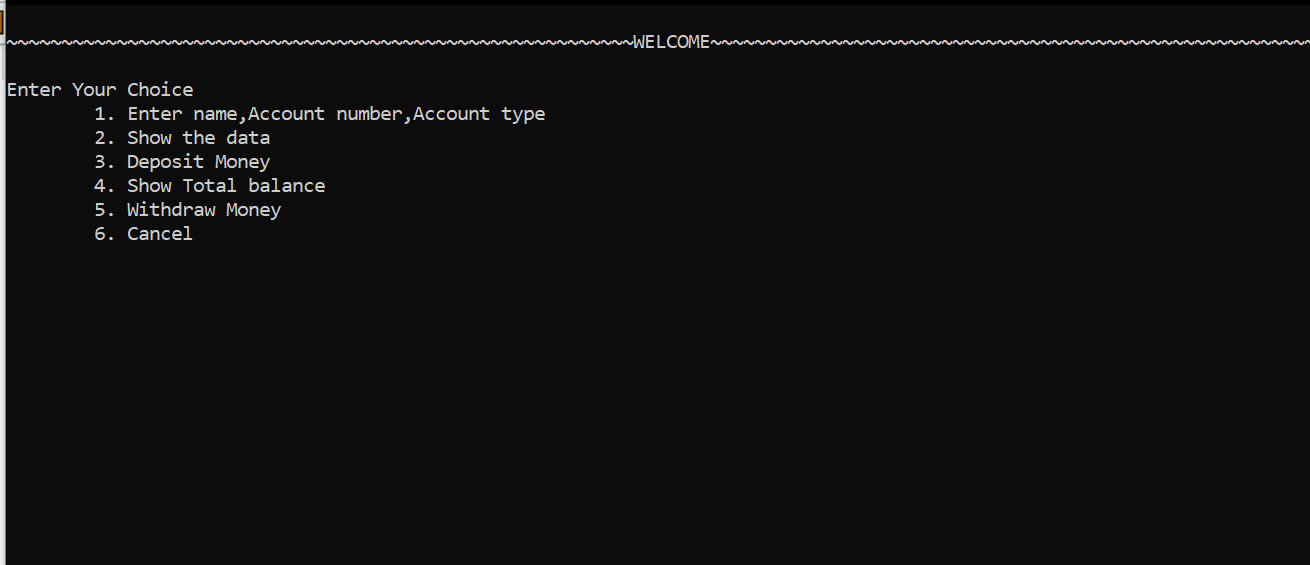• For Choice 1: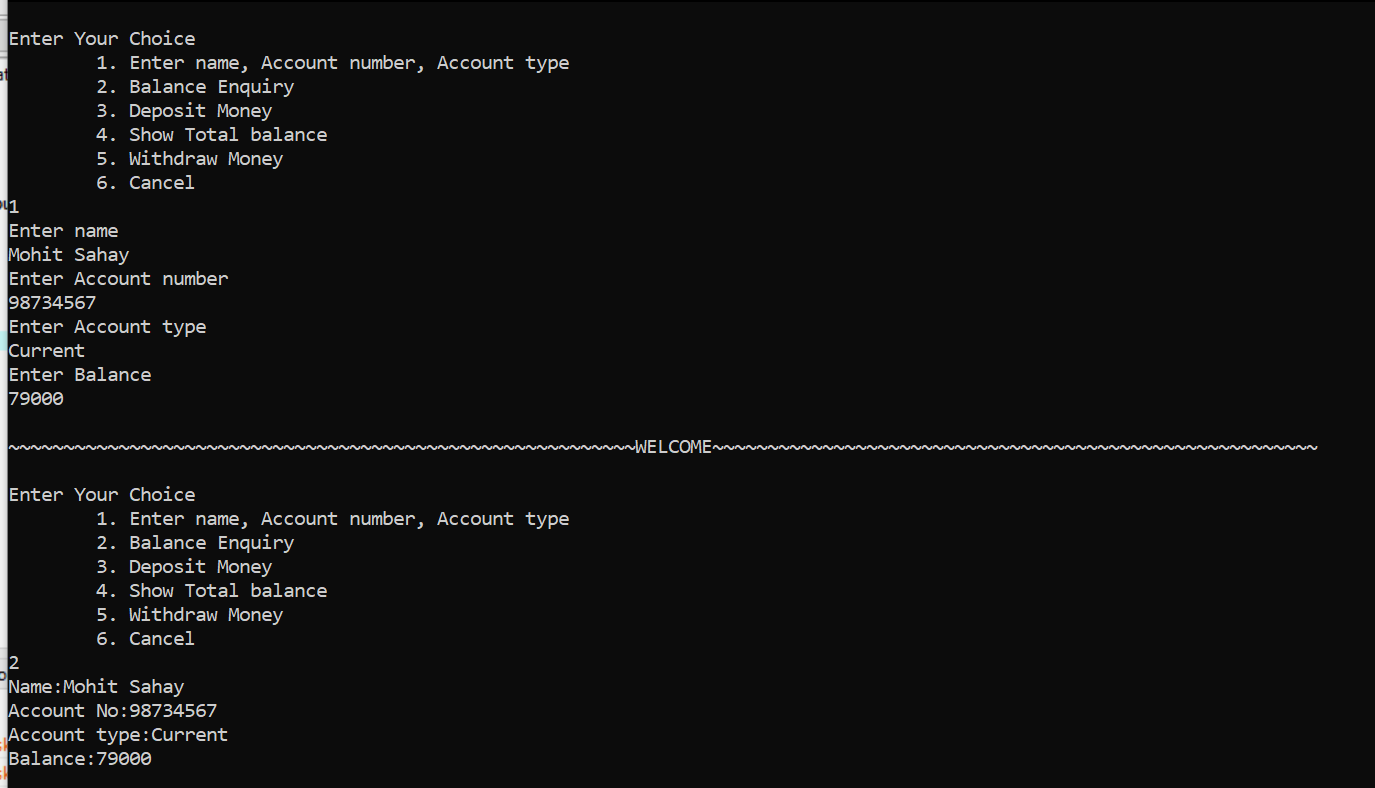• For Choice 3: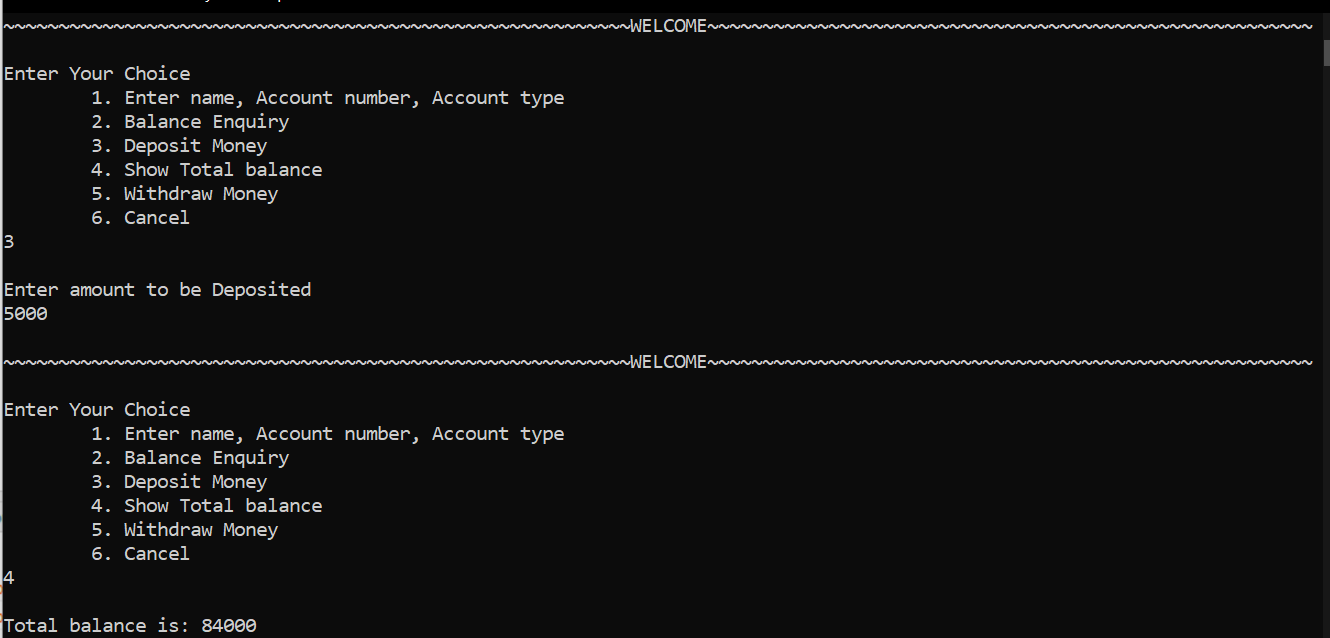• For Choice 2: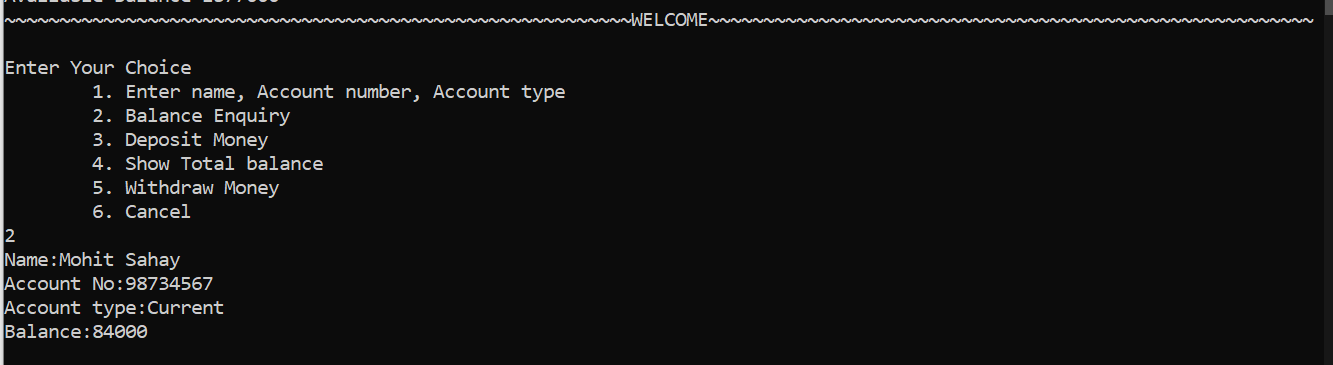• For Choice 5: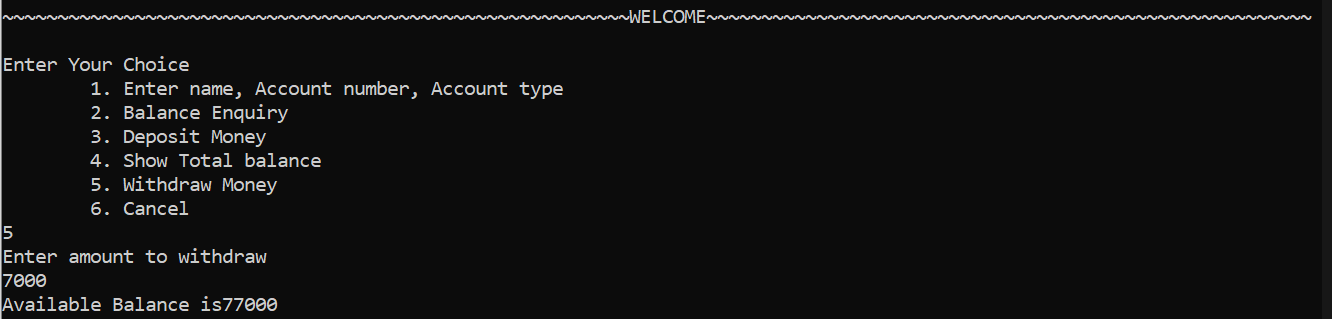My Personal Notes arrow_drop_up